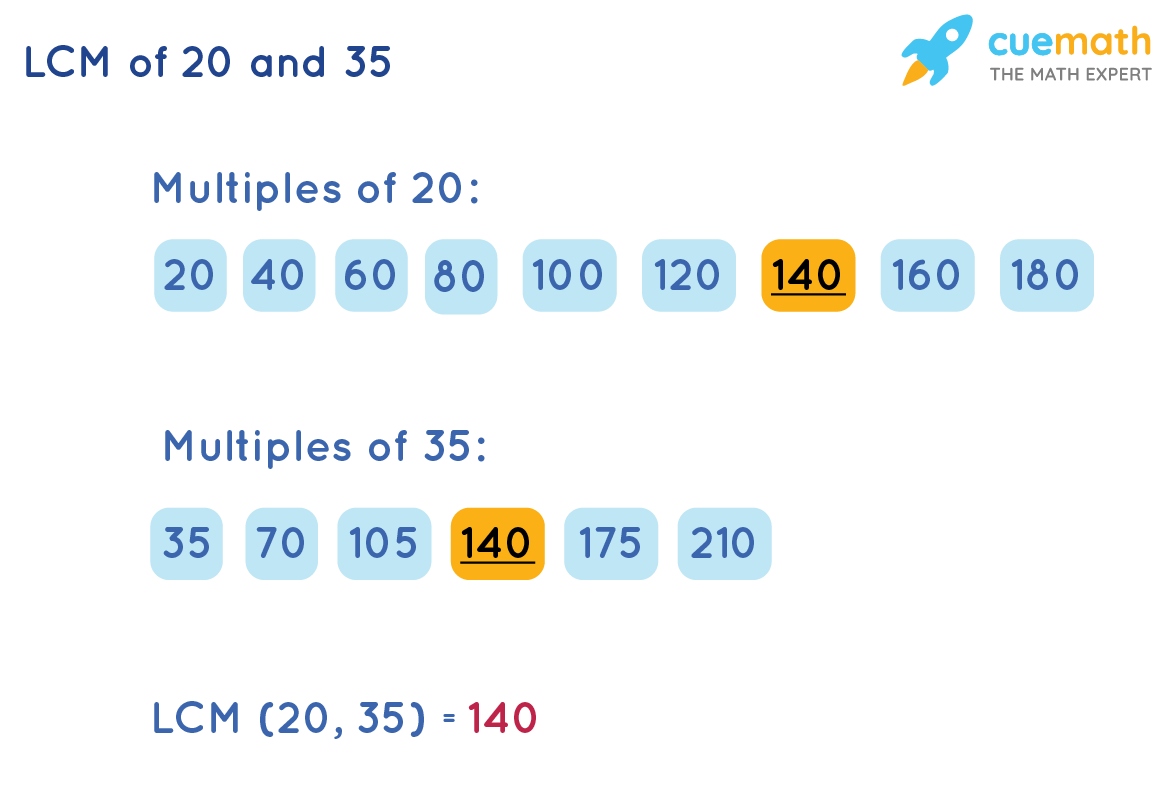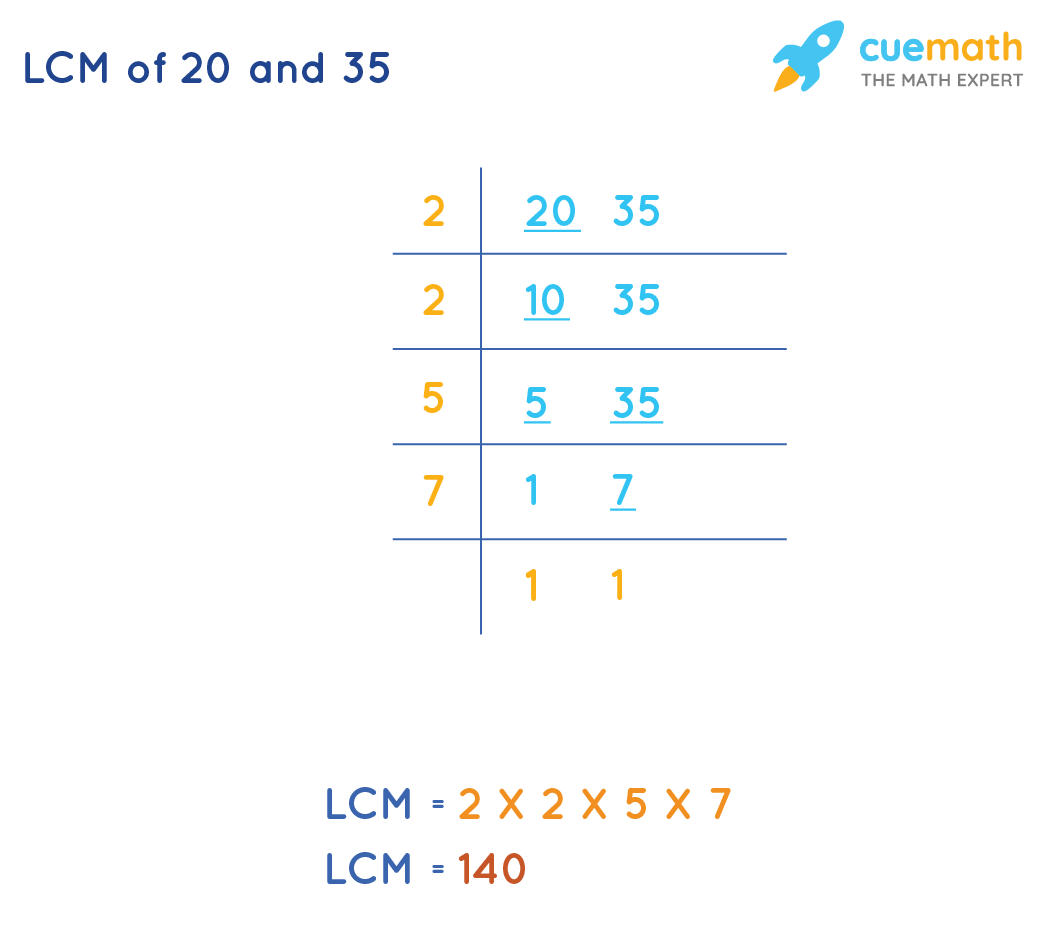# LCM of 20 and 35

## Question: What is the LCM of  20 and 35?

LCM(20, 35) is the least number which is exactly divisible by both the numbers, 20 and 35.

## Answer: LCM(20, 35) is 140

Lets see how to find the LCM of 20 and 35

## Explanation:

Least Common Multiple is the smallest number that divides both the numbers, 20 and 35 exactly.

Look at the two methods to find LCM(20, 35) explained below.

## Methods to Find LCM of 20 and 35

Let us use the following methods to find the LCM of 20 and 35

• Listing the Multiples
• Common Division

### In this method, we list the first few multiples(20, 35) and identify the common multiples.

The least among the common multiples is the LCM(20, 35)So, LCM of 20 and 35 is 140

### LCM(20, 35) by Common Division Method

In this division method, we divide the numbers simultaneously with prime numbers and stop it when we don't have a prime number to divide the given numbers.By this method, we get LCM(20, 35) = 140

Irrespective of the method, the solution to our question LCM(20, 35) is the same.